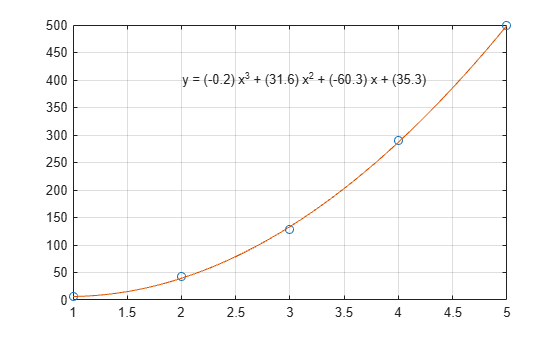# Polynomial Curve Fitting

This example shows how to fit a polynomial curve to a set of data points using the `polyfit` function. You can use `polyfit` to find the coefficients of a polynomial that fits a set of data in a least-squares sense using the syntax

```p = polyfit(x,y,n), ```

where:

• `x` and `y` are vectors containing the `x` and `y` coordinates of the data points

• `n` is the degree of the polynomial to fit

Create some x-y test data for five data points.

```x = [1 2 3 4 5]; y = [5.5 43.1 128 290.7 498.4];```

Use `polyfit` to find a third-degree polynomial that approximately fits the data.

`p = polyfit(x,y,3)`
```p = 1×4 -0.1917 31.5821 -60.3262 35.3400 ```

After you obtain the polynomial for the fit line using `polyfit`, you can use `polyval` to evaluate the polynomial at other points that might not have been included in the original data.

Compute the values of the `polyfit` estimate over a finer domain and plot the estimate over the real data values for comparison. Include an annotation of the equation for the fit line.

```x2 = 1:.1:5; y2 = polyval(p,x2); plot(x,y,'o',x2,y2) grid on s = sprintf('y = (%.1f) x^3 + (%.1f) x^2 + (%.1f) x + (%.1f)',p(1),p(2),p(3),p(4)); text(2,400,s)```